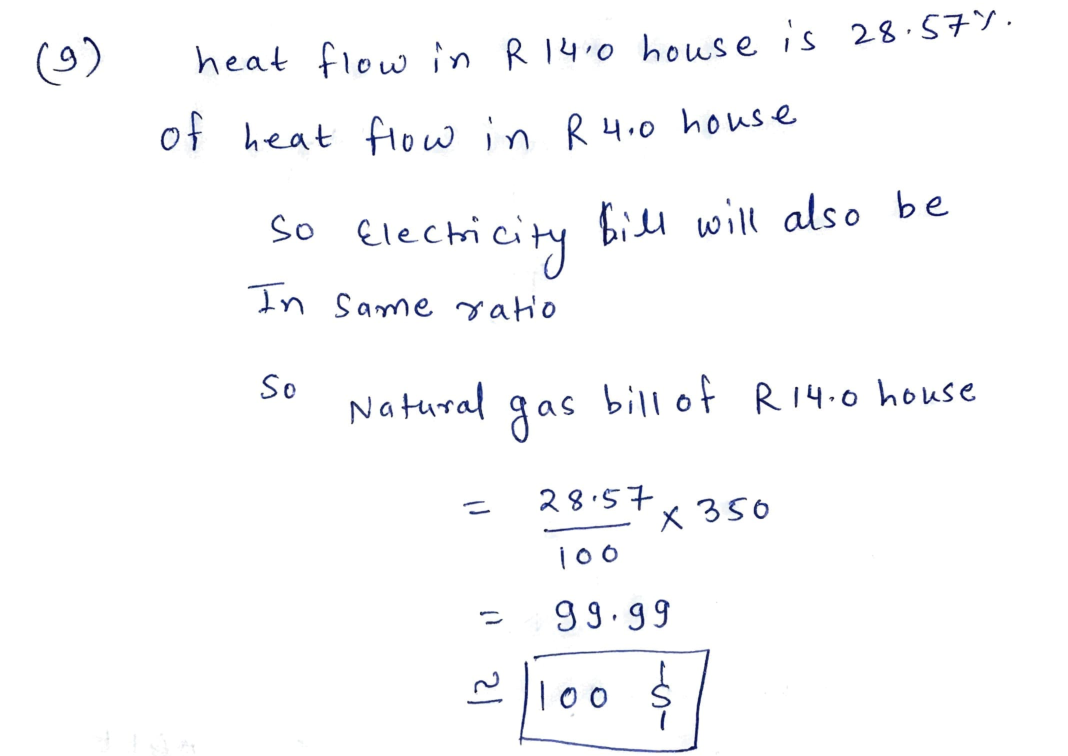Question

Renewable Energy

9. (10%) If a homeowner with an R 4.0 house paid \$350/month for his natural gas bill and the house next door had an R 14.0 house of the same area, what would you expect for the natural gas bill for the R 14.0 house?Verified### Question 36808Renewable Energy

44. Describe what is a natural frequency of a system? (3%)

### Question 36807Renewable Energy

B. Label the three damping scenarios for a SDOF system: (3%)

### Question 36806Renewable Energy

What is the equation of motion for a dynamic system best described as: (1%)
a) A homogeneous second order differential equation.
b) A time dependent algebraic equation.
c) A trigonometric transcendental equation.

### Question 36805Renewable Energy

List Newton's Laws. (3%)
Newton's Third Law:
Newton's Second Law:
Newton's First Law:

### Question 36804Renewable Energy

What is the equation of motion for a SDOF free vibration system? Define each term in the equation. (3%)

### Question 36803Renewable Energy

). Define the power coefficient for a wind turbine system: (3%)

### Question 36802Renewable Energy

Comparing VAWT and HAWAT, which one causes more obstruction to birds?

### Question 36801Renewable Energy

7. Comparing VAWT and HAWT, which one sways less? (1%)

### Question 36800Renewable Energy

6. Name the following two vertical wind turbine systems: (2%)

### Question 36799Renewable Energy

. As a result of Betz's assumption, the theoretical extractable wind power would be: (1%)
a) Larger than 60%
b) Less than 60%

### Submit query